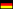Hamburg University of Technology / Institute of Mathematics / Research Topics / Observability and controllability for systems in Banach spacesObservability and controllability for systems in Banach spaces

Description

Let $$X,Y$$ be Banach spaces, $$(S_t)_{t \geq 0}$$ a $$C_0$$-semigroup on $$X$$, $$-A$$ the corresponding infinitesimal generator on $$X$$, $$C$$ a bounded operator from $$X$$ to $$Y$$ and $$T>0$$. We consider systems of the form \begin{equation} \begin{aligned} \dot{x}(t) & = -Ax(t), \quad & &t\in (0,T] ,\quad x(0) = x_0 \in X, \\ y(t) &= Cx(t), \quad & & t\in [0,T] \end{aligned} \end{equation}

and study the question whether one can reconstruct the final state $$x(T)$$ from the measurements $$y(t)$$ for $$t \in (0,T)$$. This relates to a final state observability estimate, that is, there exists $$C_{\mathrm{obs}} > 0$$ such that for all $$x_0 \in X$$ we have $$\lVert x (T) \rVert_X \leq C_{\mathrm{obs}} \lVert y \rVert_{L_r ((0,T) ; Y)}$$ for some $$r\in [1,\infty]$$.

The most studied example is the self-adjoint Schrödinger operator $$A = \Delta - V$$ in $$L_2 (\Omega)$$ with bounded potential $$V$$, and $$C = \mathrm{1}_E$$ for $$E \subset \Omega \subset \mathbb{R}^d$$. One possible approach to show an observability estimate is the so-called Lebeau-Robbiano method , that is, to prove a quantitative uncertainty relation for spectral projectors. This is an inequality of the type $\begin{equation*} \forall \lambda > 0 \ \forall \psi \in L_2 (\Omega) \colon \quad \lVert P (\lambda) \psi \rVert_{L_2 (\Omega)} \leq d_0 \mathrm{e}^{d_1 \lambda^{\gamma}} \lVert \mathrm{1}_E P (\lambda) \psi \rVert_{L_2 (E)} , \end{equation*}$

where $$\gamma \in (0,1)$$, $$d_0,d_1 > 0$$, and where $$P (\lambda)$$ denotes the projector to the spectral subspace of $$-\Delta + V$$ below $$\lambda$$. Subsequently, this strategy is generalized to semigroups in abstract Hilbert spaces. In particular the $$P(\lambda)$$ are allowed to be arbitrary projectors (onto semigroup invariant subspaces) by assuming additionally a so-called dissipation estimate, that is, a decay estimate of the semigroup on the orthogonal complement of the range of $$P(\lambda)$$.

A natural setup to ask for observability estimates is the context of Banach spaces and $$C_0$$-semigroups. In , we extend the above-mentioned stategy to the Banach space setting. In particular, we show in the general framework of Banach spaces that an uncertainty relation together with a dissipation estimate implies a final state observability estimate. Our observability constant $$C_{\mathrm{obs}}$$ is given explicitly with respect to the parameters coming from the uncertainty relation and the dissipation estimate and, in addition, is sharp in the dependence on $$T$$.

Using the well-known relation between observability and null-controllability of the predual system one can proof controllability results by the adapted Lebeau-Robbiano method. In  we consider parabolic control systems on $$L_p(\mathbb{R}^d)$$, $$p\in [1,\infty)$$, of the form $\begin{equation} \dot{x}(t) = -A_p x(t) + \mathrm{1}_E u(t),\quad t\in (0,T],\quad x(0) = x_0\in L_p(\mathbb{R}^d), \end{equation}$

where $$-A_p$$ is a strongly elliptic differential operator with constant coefficients. Assuming that $$E\subset \mathbb{R}^d$$ is a so-called thick set, we proof (approximate) null-controllability, i.e. for all $$x_0\in L_p(\mathbb{R}^d)$$ there is $$u \in L_r ((0,T);L_p (E))$$ which steers the mild solution at time $$T$$ (approximately) to zero.

A weaker concept than null-controllability is stabilizability. In the ongoing work  the uncertainty relation and the dissipation estimate in the Lebeau-Robbiano method are weakend to proof stabilizabilty for systems which are not null-controllable. ## References

 G. Lebeau and L. Robbiano. Contrôle exact de l?équation de la chaleur, Comm. Partial Differential Equations, 20(1?2):335?356, 1995.

 D. Gallaun, C. Seifert, and M. Tautenhahn. Sufficient criteria and sharp geometric conditions for observability in Banach spaces, SIAM J. Control Optim., 58(4):2639?2657, 2020.

 C. Bombach, D. Gallaun, C. Seifert, and M. Tautenhahn. Observability and null-controllability for parabolic equations in $$L_p$$-spaces. arXiv:2005.14503

 M. Egidi, D. Gallaun, C. Seifert, and M. Tautenhahn. Sufficient criteria for stabilization properties in Banach spaces. In preparation.